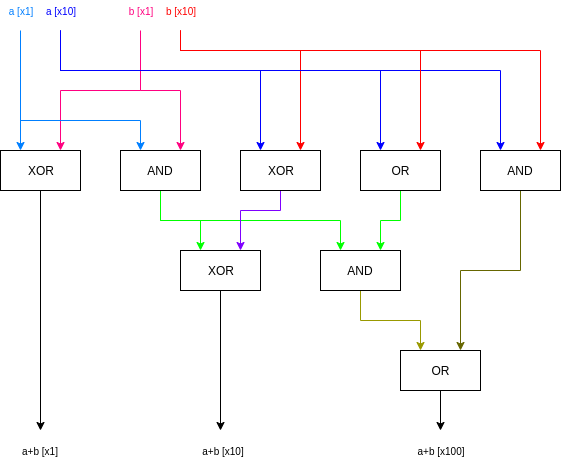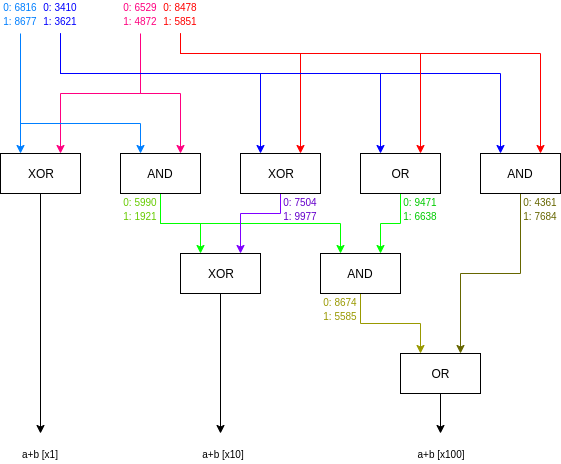# A Quick Garbled Circuits Primer

2020 Mar 21 See all posts

A Quick Garbled Circuits Primer

Special thanks to Dankrad Feist for review

Garbled circuits are a quite old, and surprisingly simple, cryptographic primitive; they are quite possibly the simplest form of general-purpose "multi-party computation" (MPC) to wrap your head around.

Here is the usual setup for the scheme:

• Suppose that there are two parties, Alice and Bob, who want to compute some function f(alice_inputs, bob_inputs), which takes inputs from both parties. Alice and Bob want to both learn the result of computing f, but Alice does not want Bob to learn her inputs, and Bob does not want Alice to learn his inputs. Ideally, they would both learn nothing except for just the output of f.
• Alice performs a special procedure ("garbling") to encrypt a circuit (meaning, a set of AND, OR... gates) which evaluates the function f. She passes along inputs, also encrypted in a way that's compatible with the encrypted circuit, to Bob.
• Bob uses a technique called "1-of-2 oblivious transfer" to learn the encrypted form of his own inputs, without letting Alice know which inputs he obtained.
• Bob runs the encrypted circuit on the encrypted data and gets the answer, and passes it along to Alice.

Extra cryptographic wrappings can be used to protect the scheme against Alice and Bob sending wrong info and giving each other an incorrect answer; we won't go into those here for simplicity, though it suffices to say "wrap a ZK-SNARK around everything" is one (quite heavy duty and suboptimal!) solution that works fine.

So how does the basic scheme work? Let's start with a circuit:This is one of the simplest examples of a not-completely-trivial circuit that actually does something: it's a two-bit adder. It takes as input two numbers in binary, each with two bits, and outputs the three-bit binary number that is the sum.

Now, let's encrypt the circuit. First, for every input, we randomly generate two "labels" (think: 256-bit numbers): one to represent that input being 0 and the other to represent that input being 1. Then we also do the same for every intermediate wire, not including the output wires. Note that this data is not part of the "garbling" that Alice sends to Bob; so far this is just setup.Now, for every gate in the circuit, we do the following. For every combination of inputs, we include in the "garbling" that Alice provides to Bob the label of the output (or if the label of the output is a "final" output, the output directly) encrypted with a key generated by hashing the input labels that lead to that output together. For simplicity, our encryption algorithm can just be enc(out, in1, in2) = out + hash(k, in1, in2) where k is the index of the gate (is it the first gate in the circuit, the second, the third?). If you know the labels of both inputs, and you have the garbling, then you can learn the label of the corresponding output, because you can just compute the corresponding hash and subtract it out.

Here's the garbling of the first XOR gate:

Inputs Output Encoding of output
00 0 0 + hash(1, 6816, 6529)
01 1 1 + hash(1, 6816, 4872)
10 1 1 + hash(1, 8677, 6529)
11 0 0 + hash(1, 8677, 4872)

Notice that we are including the (encrypted forms of) 0 and 1 directly, because this XOR gate's outputs are directly final outputs of the program. Now, let's look at the leftmost AND gate:

Inputs Output Encoding of output
00 0 5990 + hash(2, 6816, 6529)
01 0 5990 + hash(2, 6816, 4872)
10 0 5990 + hash(2, 8677, 6529)
11 1 1921 + hash(2, 8677, 4872)

Here, the gate's outputs are just used as inputs to other gates, so we use labels instead of bits to hide these intermediate bits from the evaluator.

The "garbling" that Alice would provide to Bob is just everything in the third column for each gate, with the rows of each gate re-ordered (to avoid revealing whether a given row corresponds to a 0 or a 1 in any wire). To help Bob learn which value to decrypt for each gate, we'll use a particular order: for each gate, the first row becomes the row where both input labels are even, in the second row the second label is odd, in the third row the first label is odd, and in the fourth row both labels are odd (we deliberately chose labels earlier so that each gate would have an even label for one output and an odd label for the other). We garble every other gate in the circuit in the same way.

All in all, Alice sends to Bob four ~256 bit numbers for each gate in the circuit. It turns out that four is far from optimal; see here for some optimizations on how to reduce this to three or even two numbers for an AND gate and zero (!!) for an XOR gate. Note that these optimizations do rely on some changes, eg. using XOR instead of addition and subtraction, though this should be done anyway for security.

When Bob receives the circuit, he asks Alice for the labels corresponding to her input, and he uses a protocol called "1-of-2 oblivious transfer" to ask Alice for the labels corresponding to his own input without revealing to Alice what his input is. He then goes through the gates in the circuit one by one, uncovering the output wires of each intermediate gate.

Suppose Alice's input is the two left wires and she gives (0, 1), and Bob's input is the two right wires and he gives (1, 1). Here's the circuit with labels again:• At the start, Bob knows the labels 6816, 3621, 4872, 5851
• Bob evaluates the first gate. He knows 6816 and 4872, so he can extract the output value corresponding to (1, 6816, 4872) (see the table above) and extracts the first output bit, 1
• Bob evaluates the second gate. He knows 6816 and 4872, so he can extract the output value corresponding to (2, 6816, 4872) (see the table above) and extracts the label 5990
• Bob evaluates the third gate (XOR). He knows 3621 and 5851, and learns 7504
• Bob evaluates the fourth gate (OR). He knows 3621 and 5851, and learns 6638
• Bob evaluates the fifth gate (AND). He knows 3621 and 5851, and learns 7684
• Bob evaluates the sixth gate (XOR). He knows 5990 and 7504, and learns the second output bit, 0
• Bob evaluates the seventh gate (AND). He knows 5990 and 6638, and learns 8674
• Bob evaluates the eighth gate (OR). He knows 8674 and 7684, and learns the third output bit, 1

And so Bob learns the output: 101. And in binary 10 + 11 actually equals 101 (the input and output bits are both given in smallest-to-greatest order in the circuit, which is why Alice's input 10 is represented as (0, 1) in the circuit), so it worked!

Note that addition is a fairly pointless use of garbled circuits, because Bob knowing 101 can just subtract out his own input and get 101 - 11 = 10 (Alice's input), breaking privacy. However, in general garbled circuits can be used for computations that are not reversible, and so don't break privacy in this way (eg. one might imagine a computation where Alice's input and Bob's input are their answers to a personality quiz, and the output is a single bit that determines whether or not the algorithm thinks they are compatible; that one bit of information won't let Alice or Bob know anything about each other's individual quiz answers).

### 1 of 2 Oblivious Transfer

Now let us talk more about 1-of-2 oblivious transfer, this technique that Bob used to obtain the labels from Alice corresponding to his own input. The problem is this. Focusing on Bob's first input bit (the algorithm for the second input bit is the same), Alice has a label corresponding to 0 (6529), and a label corresponding to 1 (4872). Bob has his desired input bit: 1. Bob wants to learn the correct label (4872) without letting Alice know that his input bit is 1. The trivial solution (Alice just sends Bob both 6529 and 4872) doesn't work because Alice only wants to give up one of the two input labels; if Bob receives both input labels this could leak data that Alice doesn't want to give up.

Here is a fairly simple protocol using elliptic curves:

1. Alice generates a random elliptic curve point, H.
2. Bob generates two points, P1 and P2, with the requirement that P1 + P2 sums to H. Bob chooses either P1 or P2 to be G * k (ie. a point that he knows the corresponding private key for). Note that the requirement that P1 + P2 = H ensures that Bob has no way to generate P1 and P2 such that he knows the corresponding private key for. This is because if P1 = G * k1 and P2 = G * k2 where Bob knows both k1 and k2, then H = G * (k1 + k2), so that would imply Bob can extract the discrete logarithm (or "corresponding private key") for H, which would imply all of elliptic curve cryptography is broken.
3. Alice confirms P1 + P2 = H, and encrypts v1 under P1 and v2 under P2 using some standard public key encryption scheme (eg. El-Gamal). Bob is only able to decrypt one of the two values, because he knows the private key corresponding to at most one of (P1, P2), but Alice does not know which one.

This solves the problem; Bob learns one of the two wire labels (either 6529 or 4872), depending on what his input bit is, and Alice does not know which label Bob learned.

### Applications

Garbled circuits are potentially useful for many more things than just 2-of-2 computation. For example, you can use them to make multi-party computations of arbitrary complexity with an arbitrary number of participants providing inputs, that can run in a constant number of rounds of interaction. Generating a garbled circuit is completely parallelizable; you don't need to finish garbling one gate before you can start garbling gates that depend on it. Hence, you can simply have a large multi-party computation with many participants compute a garbling of all gates of a circuit and publish the labels corresponding to their inputs. The labels themselves are random and so reveal nothing about the inputs, but anyone can then execute the published garbled circuit and learn the output "in the clear". See here for a recent example of an MPC protocol that uses garbling as an ingredient.

Multi-party computation is not the only context where this technique of splitting up a computation into a parallelizable part that operates on secret data followed by a sequential part that can be run in the clear is useful, and garbled circuits are not the only technique for accomplishing this. In general, the literature on randomized encodings includes many more sophisticated techniques. This branch of math is also useful in technologies such as functional encryption and obfuscation.